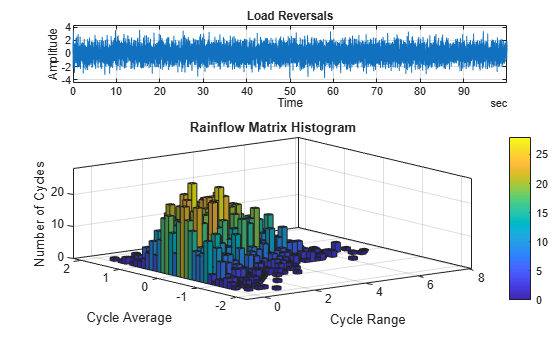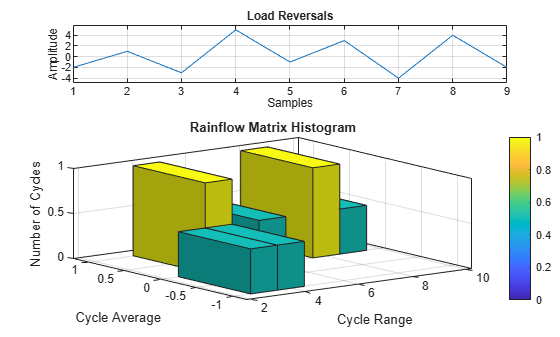# rainflow

Rainflow counts for fatigue analysis

## Syntax

``c = rainflow(x)``
``c = rainflow(x,fs)``
``c = rainflow(x,t)``
``c = rainflow(xt)``
``c = rainflow(___,'ext')``
``[c,rm,rmr,rmm] = rainflow(___)``
``[c,rm,rmr,rmm,idx] = rainflow(___)``
``rainflow(___)``

## Description

````c = rainflow(x)` returns cycle counts for the load time history, `x`, according to the ASTM E 1049 standard. See Algorithms for more information.```

example

````c = rainflow(x,fs)` returns cycle counts for `x` sampled at a rate `fs`.```

example

````c = rainflow(x,t)` returns cycle counts for `x` sampled at the time values stored in `t`.```

example

````c = rainflow(xt)` returns cycle counts for the time history stored in the MATLAB® timetable `xt`.```

example

````c = rainflow(___,'ext')` specifies the time history as a vector of identified reversals (peaks and valleys). `'ext'` can be used with any of the previous syntaxes.```
````[c,rm,rmr,rmm] = rainflow(___)` outputs a rainflow matrix, `rm`, and two vectors, `rmr` and `rmm`, containing histogram bin edges for the rows and columns of `rm`, respectively.```
````[c,rm,rmr,rmm,idx] = rainflow(___)` also returns the linear indices of the reversals identified in the input.```
````rainflow(___)` with no output arguments plots load reversals and a rainflow matrix histogram in the current figure.```

## Examples

collapse all

Generate a signal that resembles a load history, consisting of sinusoid half-periods connecting known, equispaced reversals. The signal is sampled at 512 Hz for 8 seconds. Plot the extrema and the signal.

```fs = 512; X = [-2 1 -3 5 -1 3 -4 4 -2]; lX = length(X)-1; Y = -diff(X)/2.*cos(pi*(0:1/fs:1-1/fs)') + (X(1:lX)+X(2:lX+1))/2; Y = [Y(:);X(end)]; plot(0:lX,X,'o',0:1/fs:lX,Y)```Compute cycle counts for the data. Display the matrix of cycle counts.

```[c,hist,edges,rmm,idx] = rainflow(Y,fs); T = array2table(c,'VariableNames',{'Count','Range','Mean','Start','End'})```
```T=7×5 table Count Range Mean Start End _____ _____ ____ _____ ___ 0.5 3 -0.5 0 1 0.5 4 -1 1 2 1 4 1 4 5 0.5 8 1 2 3 0.5 9 0.5 3 6 0.5 8 0 6 7 0.5 6 1 7 8 ```

Display a histogram of cycle counts as a function of stress range.

```histogram('BinEdges',edges','BinCounts',sum(hist,2)) xlabel('Stress Range') ylabel('Cycle Counts')```Use `rainflow` without output arguments to display a histogram of cycles as a function of cycle average and cycle range.

`rainflow(Y,fs)`Generate a signal that resembles a load history, consisting of sinusoid half-periods connecting known, unevenly spaced reversals. The signal is sampled at 10 Hz for 15 seconds. Plot the extrema and the signal.

```fs = 10; X = [0 1 3 4 5 6 8 10 13 15]; Y = [-2 1 -3 5 -1 3 -4 4 -2 6]; Z = []; for k = 1:length(Y)-1 x = X(k+1)-X(k); z = -(Y(k+1)-Y(k))*cos(pi*(0:1/fs:x-1/fs)/x)+Y(k+1)+Y(k); Z = [Z z/2]; end Z = [Z Y(end)]; t = linspace(X(1),X(end),length(Z)); plot(X,Y,'o',t,Z)```Compute cycle counts for the data. Display the matrix of cycle counts.

```[c,hist,edges,rmm,idx] = rainflow(Z,t); TT = array2table(c,'VariableNames',{'Count','Range','Mean','Start','End'})```
```TT=7×5 table Count Range Mean Start End _____ _____ ____ _____ ___ 0.5 3 -0.5 0 1 0.5 4 -1 1 3 1 4 1 5 6 0.5 8 1 3 4 1 6 1 10 13 0.5 9 0.5 4 8 0.5 10 1 8 15 ```

Use `rainflow` without output arguments to display a histogram of cycles as a function of cycle average and cycle range.

`rainflow(Z,t)`Generate a random signal sampled at 100 Hz for 100 seconds. Store the signal and its time information in a timetable.

```fs = 100; t = seconds(0:1/fs:100-1/fs)'; x = randn(size(t)); TT = timetable(t,x);```

Display the reversals and the rainflow matrix of the signal.

`rainflow(TT)`Generate a set of extrema resembling load reversals. Plot the data.

```X = [-2 1 -3 5 -1 3 -4 4 -2]'; plot(X) xlabel('Sample Index') ylabel('Stress')```Compute cycle counts for the data. Specify that the input consists of already identified extrema.

`[C,hist,edges] = rainflow(X,'ext');`

Display a histogram of cycle counts as a function of stress range.

```histogram('BinEdges',edges','BinCounts',sum(hist,2)) xlabel('Stress Range') ylabel('Cycle Counts')```Use `rainflow` without output arguments to display a histogram of cycles as a function of cycle average and cycle range.

`rainflow(X,'ext')`## Input Arguments

collapse all

Load time history, specified as a vector. `x` must have finite values.

Data Types: `single` | `double`

Sample rate, specified as a positive real scalar.

Data Types: `single` | `double`

Time values, specified as a vector, a `duration` array, or a `duration` scalar representing the time interval between samples.

Example: `seconds(0:1/100:1)` is a `duration` array representing 1 second of sampling at 100 Hz.

Data Types: `single` | `double` | `duration`

Load time history, specified as a timetable. `xt` must contain increasing, finite row times. The timetable must contain only one numeric data vector with finite load values.

If a timetable has missing or duplicate time points, you can fix it using the tips in Clean Timetable with Missing, Duplicate, or Nonuniform Times.

Example: `timetable(seconds(0:4)',rand(5,1))` specifies a random variable sampled at 1 Hz for 4 seconds.

Data Types: `single` | `double`

## Output Arguments

collapse all

Cycle counts, returned as a matrix. `c` contains cycle information in its columns in this order: counts, ranges, mean values, initial sample indices, and final sample indices. See Algorithms for an example. If you specify a sample rate, a time interval, or a vector of time values, then the last two columns of `c` contain initial and final cycle times. If you call `rainflow` with a timetable as input, then the last two columns express the initial and final cycle times in seconds.

Rainflow matrix. The rows of `rm` correspond to cycle range, and the columns correspond to cycle mean.

Histogram bin edges, returned as vectors. `rmr` and `rmm` contain the bin edges of the rows and columns of `rm`, respectively.

Linear indices of reversals, returned as a vector.

## Algorithms

Fatigue analysis studies how damage accumulates in an object subjected to cyclical changes in stress. The number of cycles necessary to break the object depends on the cycle amplitude. Broadband input excitation contains cycles of diverse amplitude, and the presence of hysteresis in the object has the effect of nesting some cycles within others, either completely or partially. Rainflow counting estimates the number of load change cycles as a function of cycle amplitude.

Initially, `rainflow` turns the load history into a sequence of reversals. Reversals are the local minima and maxima where the load changes sign. The function counts cycles by considering a moving reference point of the sequence, Z, and a moving ordered three-point subset with these characteristics:

1. The first and second points are collectively called Y.

2. The second and third points are collectively called X.

3. In both X and Y, the points are sorted from earlier to later in time, but are not necessarily consecutive in the reversal sequence.

4. The range of X, denoted by r(X), is the absolute value of the difference between the amplitude of the first point and the amplitude of the second point. The definition of r(Y) is analogous.

The `rainflow` algorithm is as follows:At the end, the function collects the different cycles and half-cycles and tabulates their ranges, their means, and the points at which they start and end. This information can then be used to produce a histogram of cycles.

Consider this reversal sequence:StepZReversalsThree Reversals?Yr(Y)Xr(X)r(X) < r(Y)?Z in Y?Actions
1AA, B, CYesAB3BC4NoYes
1. Count AB as ½ cycle.

3. Set Z to B.

3BB, C, DYesBC4CD8NoYes
1. Count BC as ½ cycle.

3. Set Z to C.

7CC, D, E, F, GYesEF4FG7NoNo
1. Count EF as 1 cycle.

8CC, D, GYesCD8DG9NoYes
1. Count CD as ½ cycle.

3. Set Z to D.

12DD, G, H, J, KYesHJ7JK4YesRead L.
13DD, G, H, J, K, LYesJK4KL3YesRead M.
14DD, G, H, J, K, L, MYesKL3LM5NoNo
1. Count KL as 1 cycle.

15DD, G, H, J, MYesHJ7JM5YesRead N.
16DD, G, H, J, M, NYesJM5MN1YesRead P.
17DD, G, H, J, M, N, PYesMN1NP4NoNo
1. Count MN as 1 cycle.

18DD, G, H, J, PYesHJ7JP9NoNo
1. Count HJ as 1 cycle.

19DD, G, PYesDG9GP10NoYes
1. Count DG as ½ cycle.

3. Set Z to G.

20GG, POut of data

Count GP as ½ cycle.

Now collect the results.

Cycle CountRangeMeanStartEnd
½3–0.5AB
½4–1BC
141EF
½81CD
13–0.5KL
112.5MN
170.5HJ
½90.5DG
½101GP

Compare this to the result of running `rainflow` on the sequence:

`q = rainflow([-2 1 -3 5 -1 3 -4 4 -3 1 -2 3 2 6])`
```q = 0.5000 3.0000 -0.5000 1.0000 2.0000 0.5000 4.0000 -1.0000 2.0000 3.0000 1.0000 4.0000 1.0000 5.0000 6.0000 0.5000 8.0000 1.0000 3.0000 4.0000 1.0000 3.0000 -0.5000 10.0000 11.0000 1.0000 1.0000 2.5000 12.0000 13.0000 1.0000 7.0000 0.5000 8.0000 9.0000 0.5000 9.0000 0.5000 4.0000 7.0000 0.5000 10.0000 1.0000 7.0000 14.0000```

 ASTM E1049-85(2017), "Standard Practices for Cycle Counting in Fatigue Analysis." West Conshohocken, PA: ASTM International, 2011, https://www.astm.org/cgi-bin/resolver.cgi?E1049.